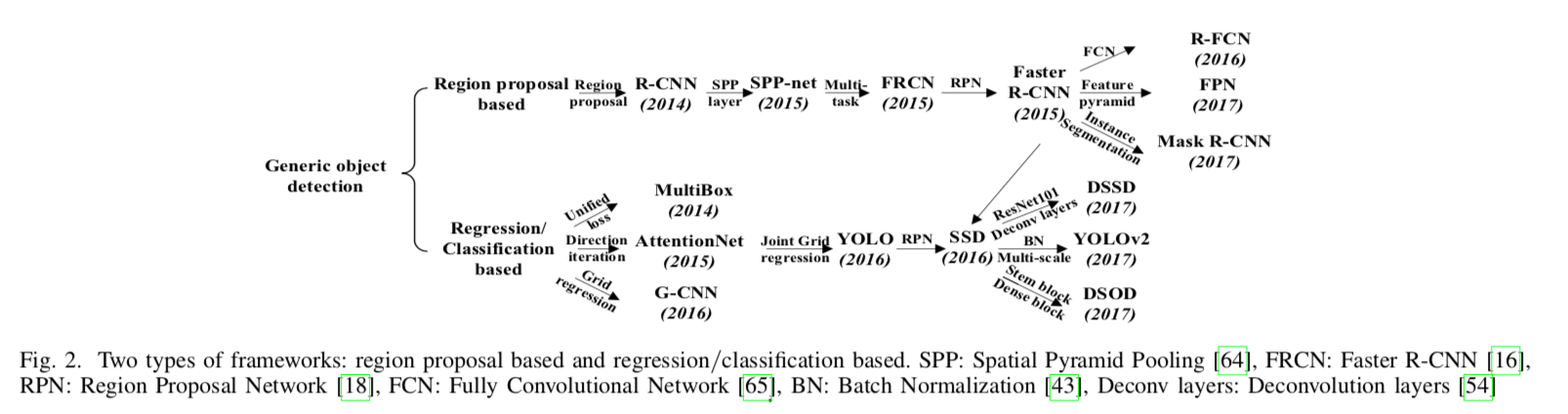### R-CNN

#### 模型框架

R-CNN的模型框架示意图如下：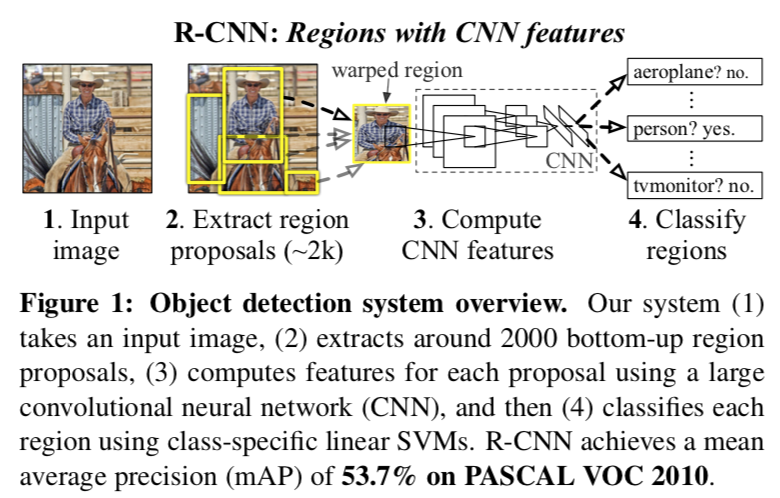##### Region proposals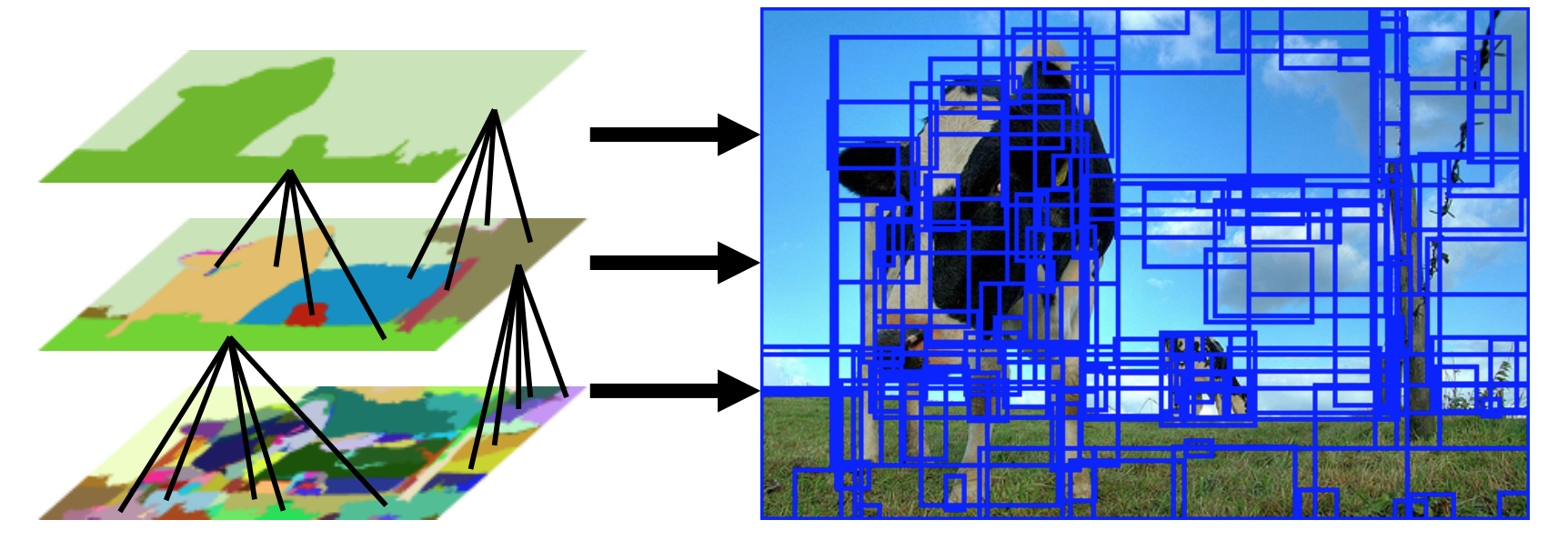Selective Search for Object Recognition

##### Feature extraction

AlexNet只接受大小固定（227×227）的输入图像，所以图像切片需要做一些转换，论文在附录A提出了几种转换方法，简单介绍如下：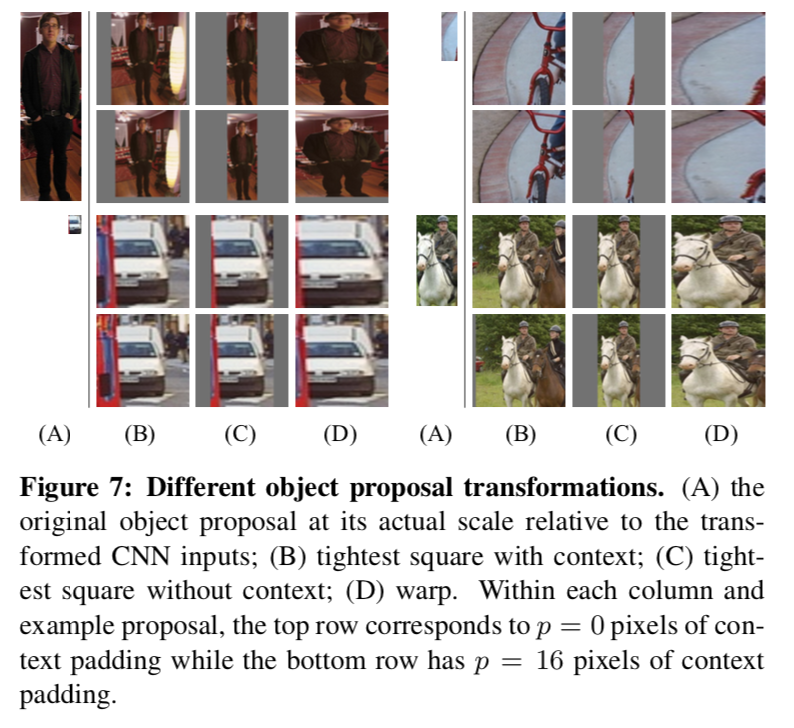4个举例种第1行和第2行的区别是边界框外延伸大小，前者不做外延，后者四周外延16（如果外延超出原图边界，填灰补充）。A列是图像切片，B列是在原图上的等比例缩放（不足填灰），C列类似B列不过不考虑原图，D列则是强制宽高缩放。论文使用的是方法D。

##### Bouding-box regression$\left\{ \begin{array}{c} t_x = (G_x-P_x)/P_w \\ t_y = (G_y-P_y)/P_h \\ t_w = log(G_w/P_w) \\ t_h = log(G_h/P_h) \end{array} \right.$

BBox回归器是在$pool_5$上接一个线性回归函数实现的，设该函数变换为$d_\star(P)=\mathbf{w}_\star^{T}\phi_5(P)$，可以通过最小二乘法求解：$\mathbf{w}_\star=\underset{\hat{\mathbf{w}}_\star}{argmin}\sum_{i}^{N}(t_\star^{i}-\hat{\mathbf{w}}_\star^{T}\phi_5(P^i))^2+\lambda\left \| \hat{\mathbf{w}}_\star \right \|^2$$\left\{ \begin{array}{c} \hat{G}_x=P_w d_x(P)+P_x \\ \hat{G}_y=P_h d_y(P)+P_y \\ \hat{G}_w=P_w exp(d_w(P)) \\ \hat{G}_h=P_h exp(d_h(P)) \end{array} \right.$

#### 实验分析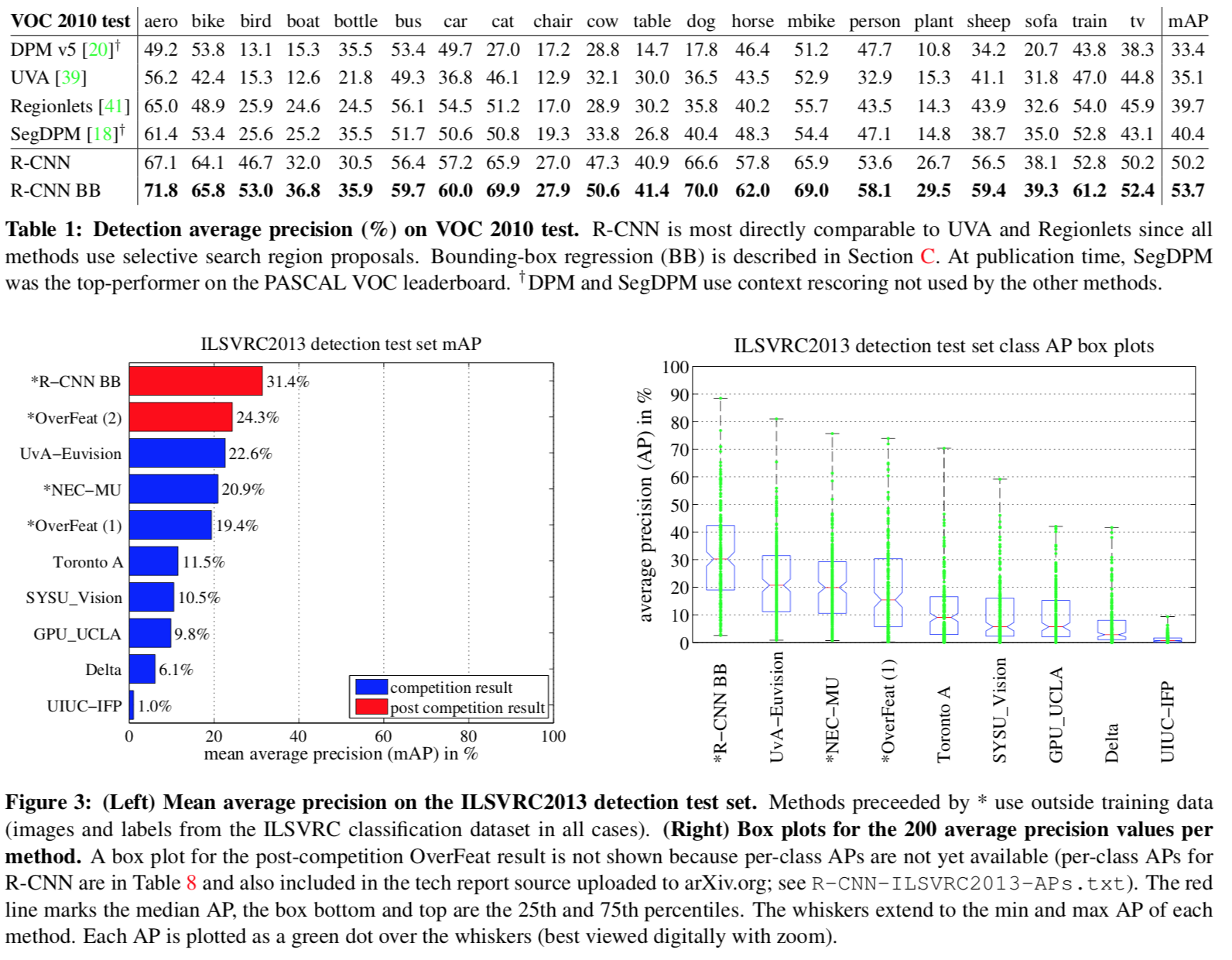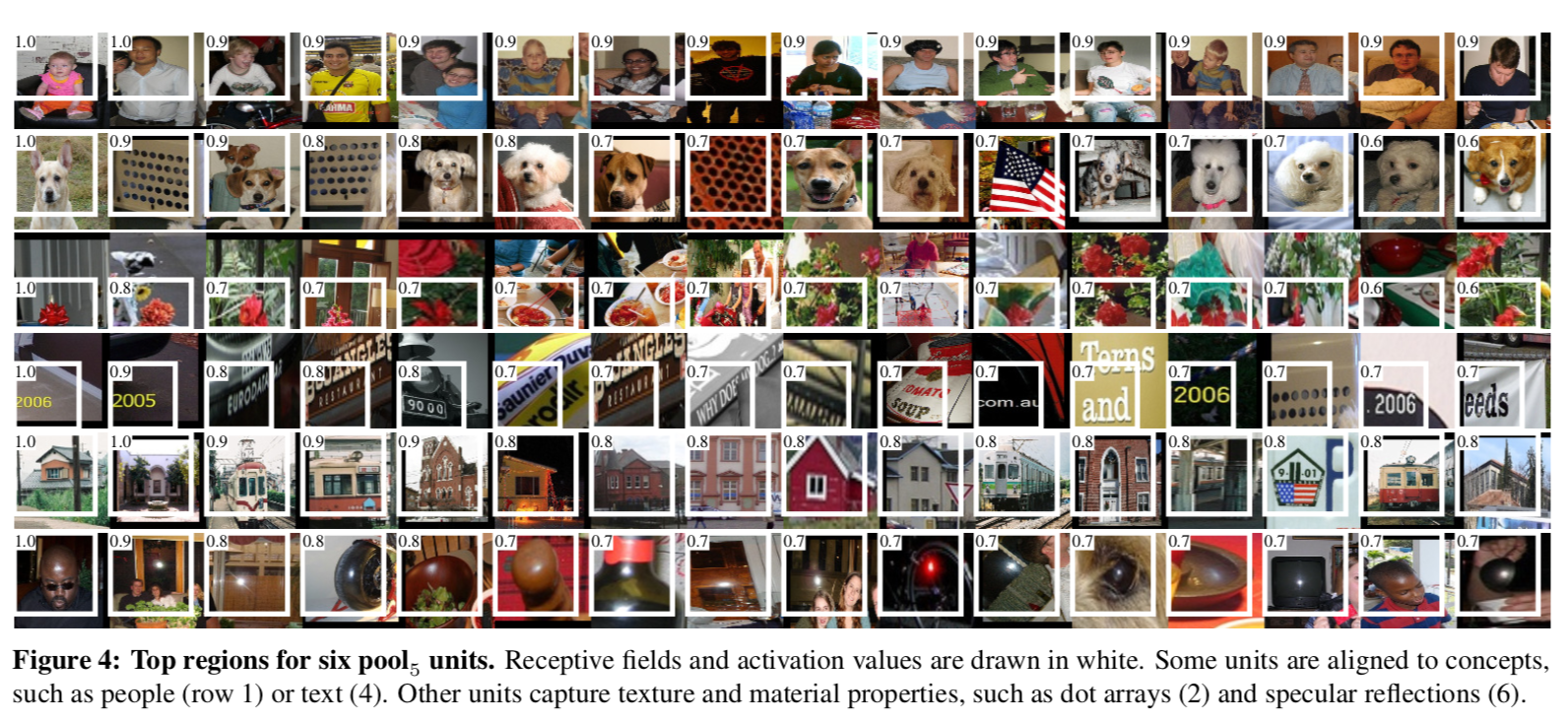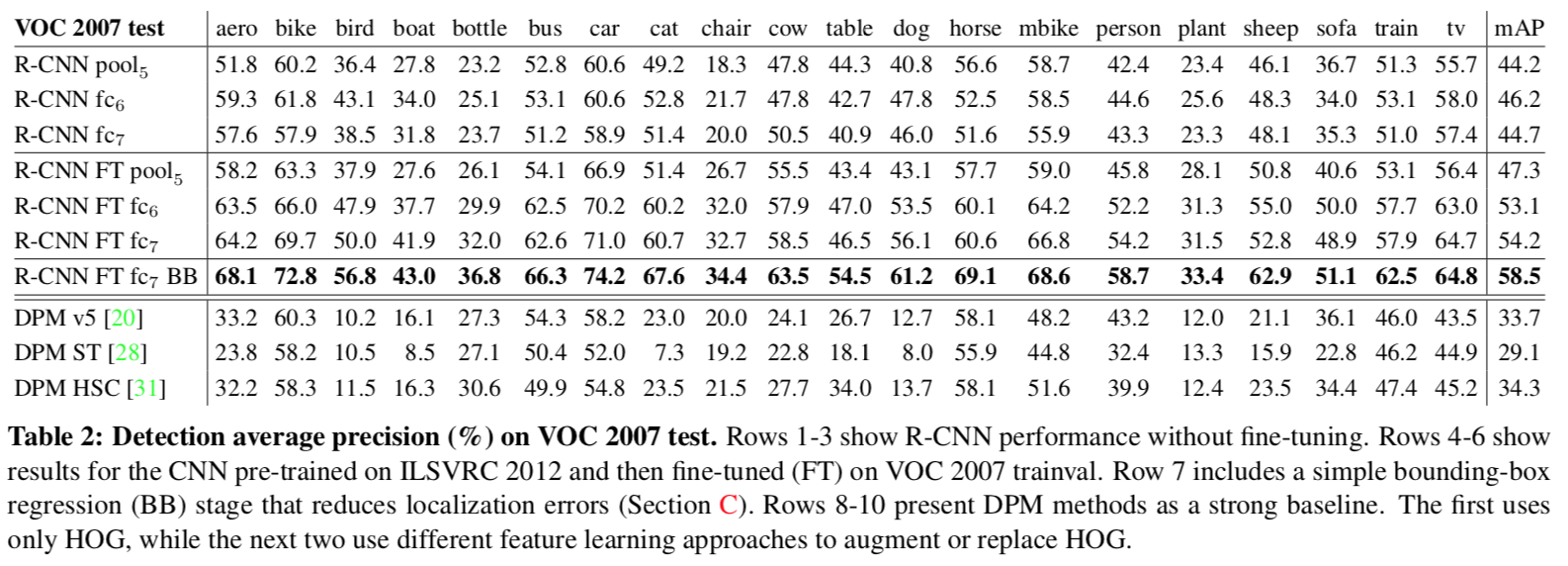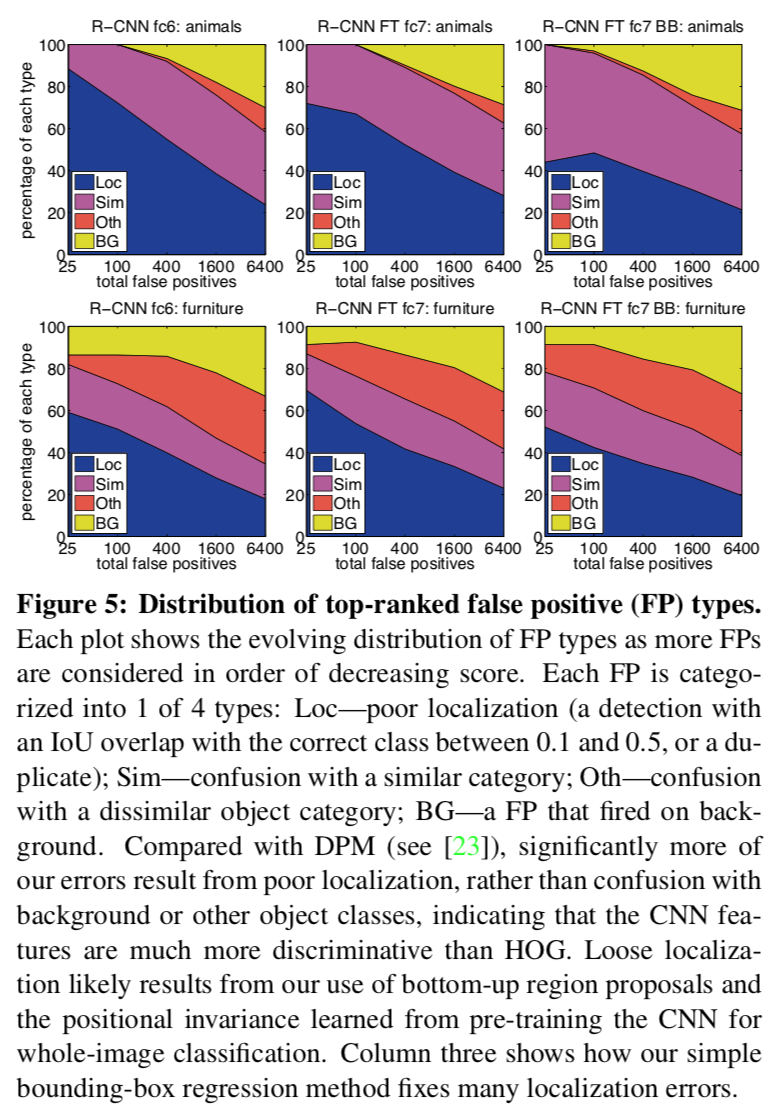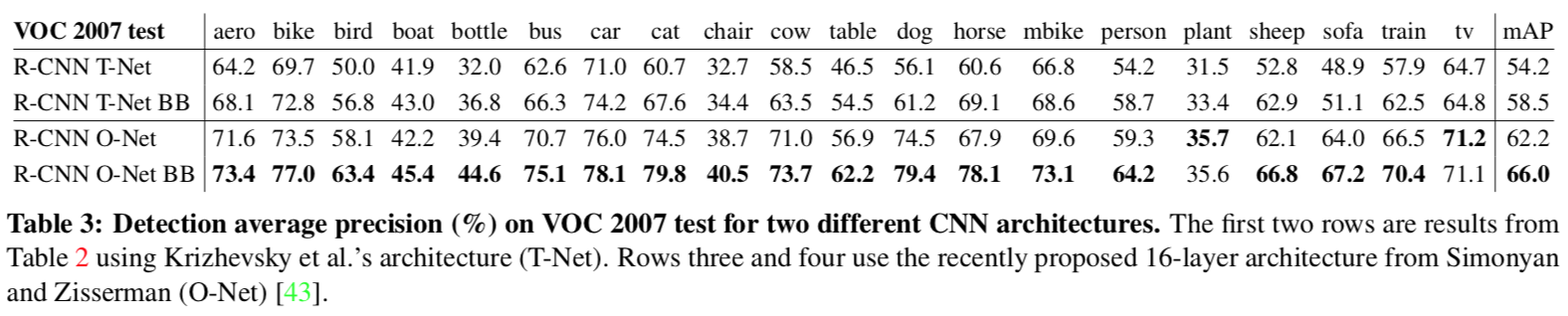#### 其他说明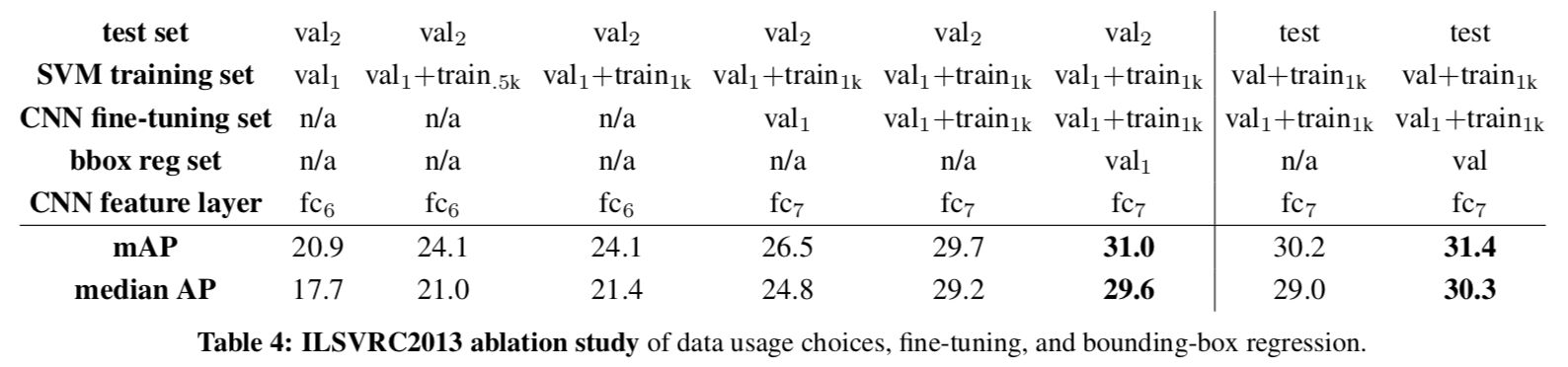val2和test上的mAP非常接近，有信心相信，val2上的mAP是测试集性能的良好指标。总的来看，数据越多越好。

### SPP-net

#### 简介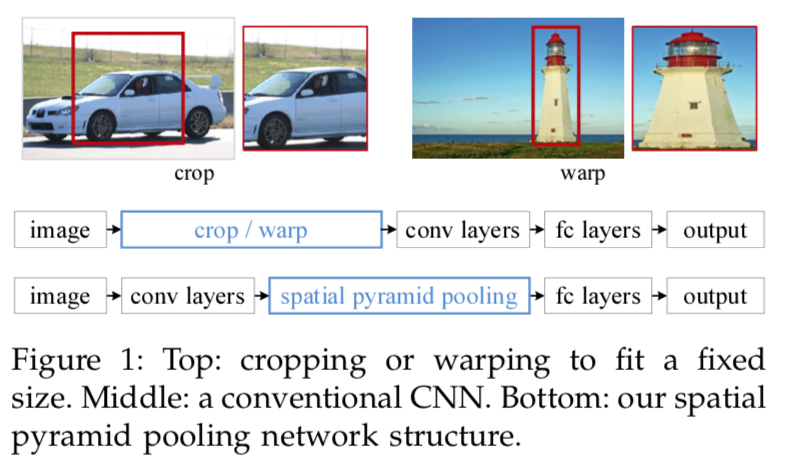#### 模型框架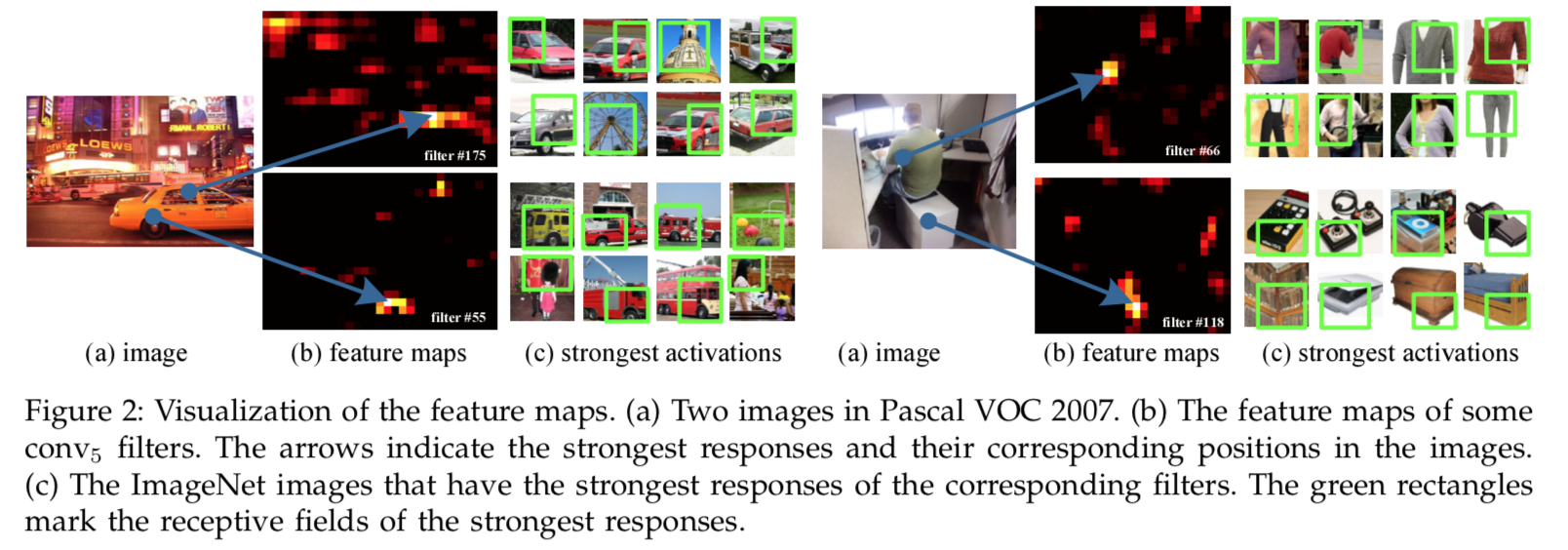SPP的做法很简单，就是在大小变化的特征图上应用数量固定的网格切分（假设划分成$n\times n$份，可以用$\lceil a/n \rceil$的window size和$\lfloor a/n \rfloor$的stride），网格内采用max-pooling，便可得到固定长度的输出特征向量，直观图解如下：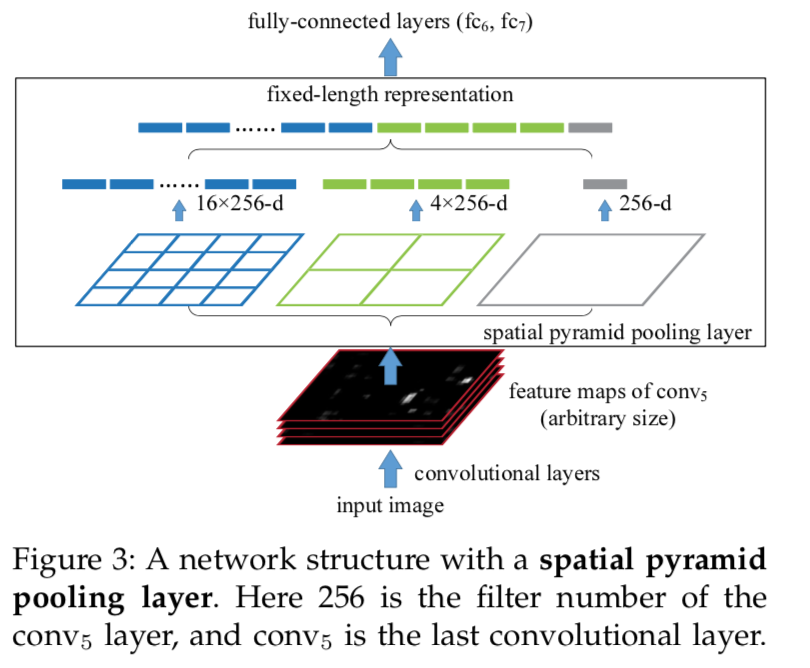1. 所有图片在输入前都做了减均值“Mean subtraction“（分通道）的预处理，看了一些资料，主要目的应该是归一化使得训练更加稳定快速；

2. 在目标检测时，原图上的区域提议框需要映射到特征图上以进行特征抽取（Mapping a Window to Feature Maps），后面的论文中通常称为RoI projection。以框的左上角$(x,y)$为例，需要在特征图上找到某点$(x',y')$使得其在原图上的感受野中心刚好落在$(x,y)$附近。

为此，我们首先回顾一下感受野的计算，其实这是前向的卷积层输出大小的逆向过程，对称公式如下：$o\_size=\frac{(i\_size + 2p - k)}{s} + 1 \\ i\_size=s(o\_size-1)+k-2p$

上面两条式子都是可以（逐层）递归进行计算的，特征图上的每个单元在原图上的感受野大小也可以通过式二计算出来。据此，可以得到感受野中心点的坐标映射公式：$p_i=s_i p_{i+1}+((k_i - 1)/2 - p)$

在SPP实现了对此作了简化，令每一层的$p$（padding）为$\lfloor k_i / 2\rfloor$，如此一来当$k_i$为奇数是（通常也是这样设定的），上式简化为$p_i=s_ip_{i+1}$$k_i$为偶数时，$p_i=s_ip_{i+1}-0.5$，因为需要取整，也可以简单近似）。

回到最开始的问题，$x$$x'$的关系经由上述分析可得$x=Sx',\ S=\prod_{0}^{i}s_i$，因此$x'$的取值为$\lfloor x/S \rfloor + 1$ （左上角）或$\lceil x/S \rceil - 1$（右下角），这里的$\pm$主要是因为感受野会有边沿放大现象，所以适当的将映射坐标往内收缩一下。

注：本部分内容参考了https://zhuanlan.zhihu.com/p/24780433

#### 实验分析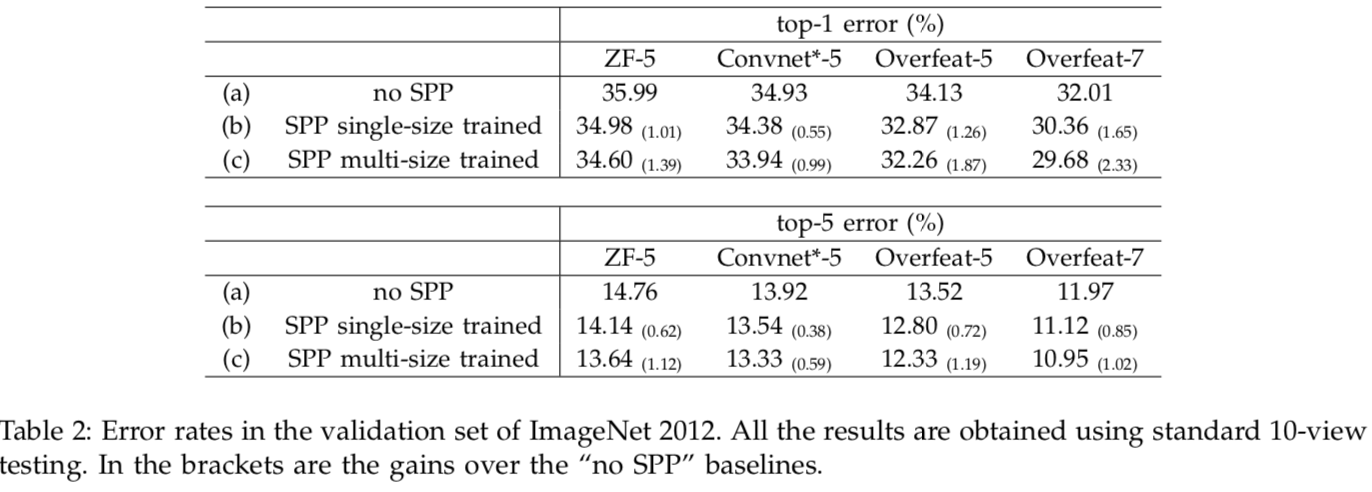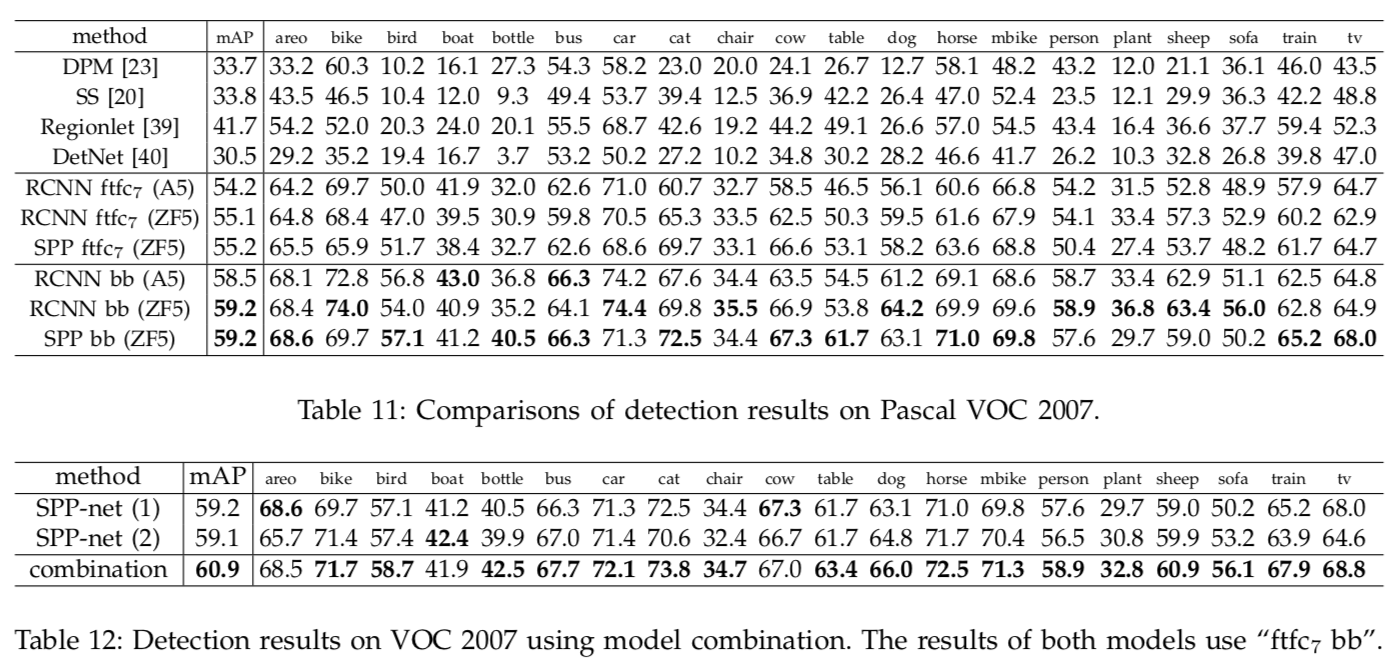### Fast R-CNN

#### 简介

SPP主要对问题2&3进行了优化，使得提议区域的特征提取时间大幅降低。但是，问题1仍旧存在，多阶段间的独立性带来了不少限制。

#### 模型框架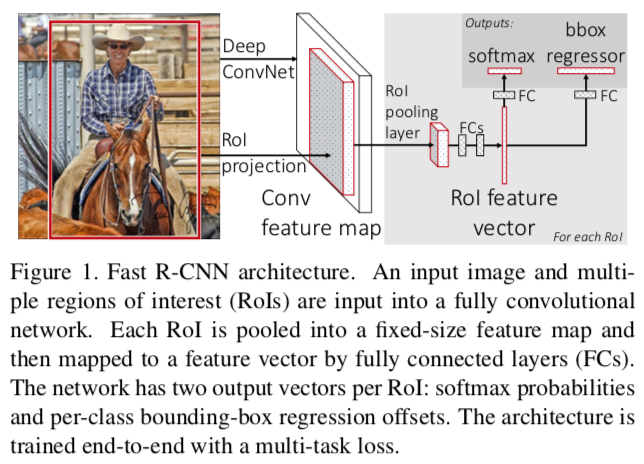RoI projection在上一节中已经介绍过，RoI pooling思想也极为简单，可以认为是单层次的SPP，即将RoI window划分为固定的$H\times W$份，然后max-pooling。$L(p,u,t^u,v)=L_{cls}(p,u)+\lambda [u\ge 1]L_{loc}(t^{u},v) \\ L_cls(p,u)=-\log p_u \\ L_loc(t^u,v)=\sum_{i\in \{x,y,w,h\}} \mathrm{smooth}_{L_1}(t_i^u-v_i) \\ \mathrm{smooth}_{L_1}(x)=\begin{cases} 0.5x^2 & \text{ if } \left | x \right | \textless 1 \\ \left | x \right | - 0.5 & \text{ otherwise } \end{cases}$

#### 实验分析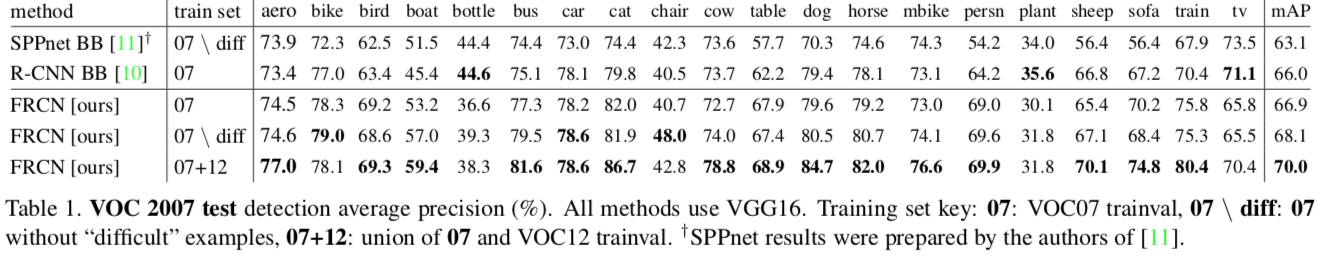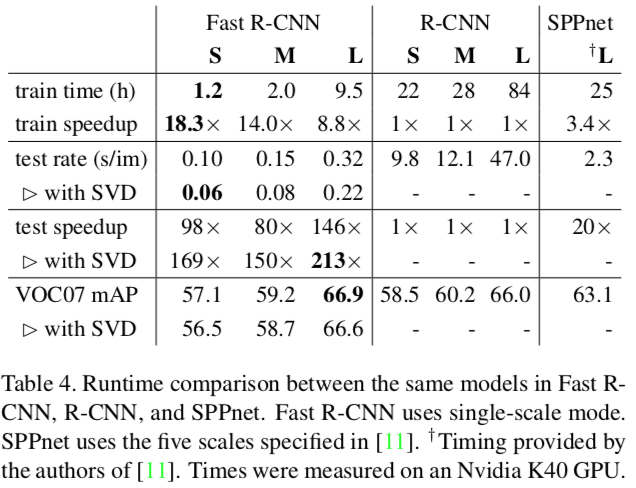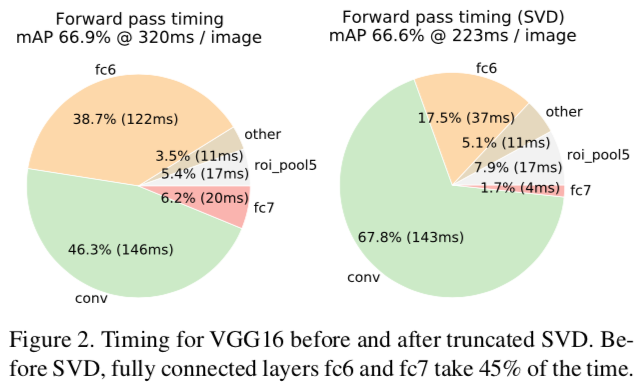S/M/L表示几种规模的基础模型 truncate SVD对性能有一些影响，但是减少了很多时间

### Reference